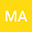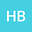loading page

Some new quantum Trapezoid and quantum Midpoint type inequalities for preinvex functions
••Hüseyin BUDAK
Düzce University
Author Profile## Abstract

In this article, we derive Hermite-Hadamard inequalities for preinvex functions using the quantum integrals and show their validation with mathematical examples. We prove midpoint and trapezoidal inequalities for q^{ϰ₂}-differentiable preinvex functions via q^{ϰ₂}-quantum integrals. Moreover, we show with an example that the already proved inequality of Hermite-Hadamard type for preinvex functions via q_{ϰ₁}-quantum integrals is not valid for preinvex functions and we give its correct version. We prove the midpoint inequalities for q_{ϰ₁}-differentiable preinvex functions via q_{ϰ₁}-quantum integrals. It is also shown that the newly proved results transformed into some known results by considering the limit q→1⁻ and η(ϰ₂,ϰ₁)=-η(ϰ₁,ϰ₂)=ϰ₂-ϰ₁ in the newly derived results.